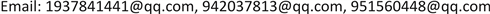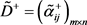﻿ 基于TOPSIS的区间三角模糊集多属性群决策方法 New Group Decision Making Method in Interval-Triangular Fuzzy Setting Based on TOPSIS

Vol. 07  No. 10 ( 2018 ), Article ID: 27206 , 12 pages
10.12677/AAM.2018.710141

New Group Decision Making Method in Interval-Triangular Fuzzy Setting Based on TOPSIS

Min Li, Bianping Su, Qiangqiang Zhang

College of Mathematics, College of Science, Xi’an University of Architecture and Technology, Xi’an ShaanxiReceived: Oct. 1st, 2018; accepted: Oct. 15th, 2018; published: Oct. 22nd, 2018ABSTRACT

In multiple attribute group decision making, because every expert has his own knowledge and expertise, different experts have different weights for different attributes. A new method to determine the expert’s weights is put forward based on the TOPSIS method in interval-triangular fuzzy setting. If the evaluation value is close to the positive idea evaluation value and far away from the negative ideal evaluation value, it will be given a large weight; otherwise, the evaluation value will be given a small weight. Experience shows that the weight of experts determined by this method has a significant effect on solving practical decision-making problems. A new method of multiple attribute interval-valued intuitionistic fuzzy group decision making is presented in this paper, including the attribute weights which are completely known, partly known and completely unknown. Finally, the feasibility and validity of this method are proved by our examples.

Keywords:Multiple Attribute Group Decision Making, Interval-Triangular Fuzzy Set, Weight, TOPSIS1. 引言

Hwang和Yoon于1981年提出了TOPSIS方法  ，并且被广泛地研究与应用，在TOPSIS方法中，一个理想方案的选择应该在接近正理想方案的同时远离负理想方案。Yang Wei等提出了一种用TOPSIS   确定专家权重的新方法，不过其中决策者给出的评价值是以直觉模糊数的形式给出的，这样对于处理决策过程中的模糊性和不确定性有一定的弊端。因此，在本文中，我们对上述方法进行了改进，在决策者的评价值是以区间三角模糊数给出的情况下，给出了一种用TOPSIS确定专家权重的一种新方法。该方法更有利于考虑到决策过程中的模糊性和不确定性，而且适用于方案集和属性集非常大的情况。为了将不同的决策者给出的评价值集结为一个，我们首先定义了区间三角模糊正，负理想矩阵，然后计算每个评价值到区间三角模糊正，负理想矩阵之间的距离，接着我们通过计算得到了每个评价值的贴近度，进一步求得了专家权重。通过我们的方法确定的权重有两个优势：第一如果评价值接近正理想点并且同时远离负理想点就会被赋予一个较高的权重；否则，评价值就会被赋予一个小的权重；第二，降低了过高或过低的评价值对排序结果的影响。并且考虑了不同属性权重信息的情况：属性权重完全已知，部分已知和完全未知。如果属性权重完全已知，在将不同的决策者给出的评价值集结为一个之后，我们用TOPSIS方法对决策结果进行排序；如果属性权重部分已知，我们通过求解一个线性规划模型来求得属性权重；如果属性权重完全未知，我们同样用TOPSIS方法得到属性权重。最后我们提出了一种与之相一致的算法，并且通过一个实例证明了该方法的有效性。

2. 区间三角模糊集基本理论

1) $\stackrel{˜}{x}+\stackrel{˜}{z}=\left[\left({x}_{1}+{z}_{1},{{x}^{\prime }}_{1}+{{z}^{\prime }}_{1}\right);{x}_{2}+{z}_{2};\left({{x}^{\prime }}_{3}+{{z}^{\prime }}_{3},{x}_{3}+{z}_{3}\right)\right]$

2) $k\stackrel{˜}{x}=\left[\left(k{x}_{1},k{{x}^{\prime }}_{1}\right);k{x}_{2};\left(k{{x}^{\prime }}_{3},k{x}_{3}\right)\right],k>0$

${M}^{*}\left(\stackrel{˜}{x}\right)={\int }_{0}^{1}\alpha \left[{x}_{3}+\left({x}_{2}-{x}_{3}\right)\alpha \right]\text{d}\alpha +{\int }_{0}^{1}\beta \left[{{x}^{\prime }}_{3}+\left({x}_{2}-{{x}^{\prime }}_{3}\right)\beta \right]\text{d}\beta =\frac{{x}_{3}+4{x}_{2}+{{x}^{\prime }}_{3}}{6}$ (1)

${M}_{*}\left(\stackrel{˜}{x}\right)={\int }_{0}^{1}\alpha \left[{x}_{1}+\left({x}_{2}-{x}_{1}\right)\alpha \right]\text{d}\alpha +{\int }_{0}^{1}\beta \left[{{x}^{\prime }}_{1}+\left({x}_{2}-{{x}^{\prime }}_{1}\right)\beta \right]\text{d}\beta =\frac{{x}_{1}+4{x}_{2}+{{x}^{\prime }}_{1}}{6}$ (2)

$P\left(\stackrel{˜}{x}>\stackrel{˜}{z}\right)=\mathrm{min}\left\{\mathrm{max}\left(\frac{{M}^{*}\left(\stackrel{˜}{x}\right)-{M}_{*}\left(\stackrel{˜}{z}\right)}{{M}^{*}\left(\stackrel{˜}{x}\right)-{M}_{*}\left(\stackrel{˜}{x}\right)+{M}^{*}\left(\stackrel{˜}{z}\right)-{M}_{*}\left(\stackrel{˜}{z}\right)},0\right),1\right\}=\left\{\begin{array}{l}1,\text{ }\text{ }\text{ }\text{ }\text{ }\text{ }\stackrel{˜}{x}>\stackrel{˜}{z}\\ 0\text{ }\text{ }\text{ }\text{ }\text{ }\text{ }\text{ }\text{ }\stackrel{˜}{x}\le \stackrel{˜}{z}\end{array}$ (3)

$\stackrel{˜}{x}>\stackrel{˜}{z}$ 的可能度。

1) $0\le P\left(\stackrel{˜}{x}>\stackrel{˜}{z}\right)\le 1$

2) $P\left(\stackrel{˜}{x}>\stackrel{˜}{z}\right)=1$ ，当且仅当 ${M}^{*}\left(\stackrel{˜}{z}\right)\le {M}_{*}\left(\stackrel{˜}{x}\right)$

3) $P\left(\stackrel{˜}{x}>\stackrel{˜}{z}\right)=0$ ，当且仅当 ${M}^{*}\left(\stackrel{˜}{x}\right)\le {M}_{*}\left(\stackrel{˜}{z}\right)$

$d\left(\stackrel{˜}{x},\stackrel{˜}{z}\right)=\frac{1}{6}\left(|{x}_{1}-{z}_{1}|+|{{x}^{\prime }}_{1}-{{z}^{\prime }}_{1}|+2|{x}_{2}-{z}_{2}|+|{{x}^{\prime }}_{3}-{{z}^{\prime }}_{3}|+|{x}_{3}-{z}_{3}|\right)$ (4)

3. 一个新的区间三角模糊集多属性群决策方法

${D}^{\left(k\right)}={\left({\stackrel{˜}{\alpha }}_{ij}^{\left(k\right)}\right)}_{m×n}=\left[\begin{array}{cccc}{\stackrel{˜}{\alpha }}_{11}^{\left(k\right)}& {\stackrel{˜}{\alpha }}_{12}^{\left(k\right)}& \cdots & {\stackrel{˜}{\alpha }}_{1n}^{\left(k\right)}\\ {\stackrel{˜}{\alpha }}_{21}^{\left(k\right)}& {\stackrel{˜}{\alpha }}_{22}^{\left(k\right)}& \cdots & {\stackrel{˜}{\alpha }}_{2n}^{\left(k\right)}\\ ⋮& ⋮& \ddots & ⋮\\ {\stackrel{˜}{\alpha }}_{n1}^{\left(k\right)}& {\stackrel{˜}{\alpha }}_{n2}^{\left(k\right)}& \cdots & {\stackrel{˜}{\alpha }}_{nn}^{\left(k\right)}\end{array}\right]$ (5)(6)

${D}_{d}^{-}={\left({\stackrel{˜}{\alpha }}_{ij}^{d}\right)}_{m×n}$ (7)

${D}_{u}^{-}={\left({\stackrel{˜}{\alpha }}_{ij}^{u}\right)}_{m×n}$ (8)

${\stackrel{˜}{\alpha }}_{ij}^{\left(k\right)}=\left[\left({a}_{ij1}^{\left(k\right)},{{a}^{\prime }}_{ij1}^{\left(k\right)}\right),{a}_{ij2}^{\left(k\right)},\left({{a}^{\prime }}_{ij3}^{\left(k\right)},{a}_{ij3}^{\left(k\right)}\right)\right]$${\stackrel{˜}{\alpha }}_{ij}^{+}=\left[\left({a}_{ij1}^{+},{{a}^{\prime }}_{ij1}^{+}\right),{a}_{ij2}^{+},\left({{a}^{\prime }}_{ij3}^{+},{a}_{ij3}^{+}\right)\right]$${\stackrel{˜}{\alpha }}_{ij}^{d}=\left[\left({a}_{ij1}^{d},{{a}^{\prime }}_{ij1}^{d}\right),{a}_{ij2}^{d},\left({{a}^{\prime }}_{ij3}^{d},{a}_{ij3}^{d}\right)\right]$${\stackrel{˜}{\alpha }}_{ij}^{u}=\left[\left({a}_{ij1}^{u},{{a}^{\prime }}_{ij1}^{u}\right),{a}_{{}_{ij2}}^{u},\left({{a}^{\prime }}_{ij3}^{u},{a}_{ij3}^{u}\right)\right]$ 之间的距离定义如下：

${d}_{ij}^{+}=\frac{1}{6}\left(|{a}_{ij1}^{\left(k\right)}-{a}_{ij1}^{+}|+|{{a}^{\prime }}_{ij1}^{\left(k\right)}-{{a}^{\prime }}_{ij1}^{+}|+2|{a}_{ij2}^{\left(k\right)}-{a}_{ij2}^{+}|+|{{a}^{\prime }}_{ij3}^{\left(k\right)}-{{a}^{\prime }}_{ij3}^{+}|+|{a}_{ij3}^{\left(k\right)}-{a}_{ij3}^{+}|\right)$ (9)

${d}_{ij}^{d}=\frac{1}{6}\left(|{a}_{ij1}^{\left(k\right)}-{a}_{ij1}^{d}|+|{{a}^{\prime }}_{ij1}^{\left(k\right)}-a{\text{'}}_{ij1}^{d}|+2|{a}_{ij2}^{\left(k\right)}-{a}_{ij2}^{d}|+|{{a}^{\prime }}_{ij3}^{\left(k\right)}-{{a}^{\prime }}_{ij3}^{d}|+|{a}_{ij3}^{\left(k\right)}-{a}_{ij3}^{d}|\right)$ (10)

${d}_{ij}^{u}=\frac{1}{6}\left(|{a}_{ij1}^{\left(k\right)}-{a}_{ij1}^{u}|+|{{a}^{\prime }}_{ij1}^{\left(k\right)}-{{a}^{\prime }}_{ij1}^{u}|+2|{a}_{ij2}^{\left(k\right)}-{a}_{ij2}^{u}|+|{{a}^{\prime }}_{ij3}^{\left(k\right)}-{{a}^{\prime }}_{ij3}^{u}|+|{a}_{ij3}^{\left(k\right)}-{a}_{ij3}^{u}|\right)$ (11)

${\stackrel{˜}{\alpha }}_{ij}^{\left(k\right)}$ 的贴近度为：

${c}_{ij}^{\left(k\right)}=\frac{{d}_{ij}^{u}+{d}_{ij}^{d}}{{d}_{ij}^{u}+{d}_{ij}^{d}+{d}_{ij}^{+}},\text{\hspace{0.17em}}\text{\hspace{0.17em}}i=1,2,\cdots ,m;j=1,2,\cdots ,n;k=1,2,\cdots ,t$ (12)

${\omega }_{ij}^{\left(k\right)}=\frac{{c}_{ij}^{\left(k\right)}}{{\sum }_{k=1}^{t}{c}_{ij}^{\left(k\right)}},\text{\hspace{0.17em}}\text{\hspace{0.17em}}i=1,2,\cdots ,m;j=1,2,\cdots ,n;k=1,2,\cdots ,t$ (13)

${\stackrel{˜}{\alpha }}_{ij}={\omega }_{ij}^{\left(1\right)}{\stackrel{˜}{\alpha }}_{ij}^{\left(1\right)}+{\omega }_{ij}^{\left(2\right)}{\stackrel{˜}{\alpha }}_{ij}^{\left(2\right)}+\cdots +{\omega }_{ij}^{\left(t\right)}{\stackrel{˜}{\alpha }}_{ij}^{\left(t\right)}$ (14)

$D={\left({\stackrel{˜}{\alpha }}_{ij}\right)}_{m×n}$ (15)

1) $\left\{{\omega }_{i}\ge {\omega }_{j}\right\},i\ne j$ ；2) $\left\{{\omega }_{i}-{\omega }_{j}\ge {\epsilon }_{i}\left(>0\right)\right\},i\ne j$

3) $\left\{{\omega }_{i}\ge {\alpha }_{i}{\omega }_{i}\right\},0\le {\alpha }_{i}\le 1,i\ne j$

4) $\left\{{\beta }_{i}\le {\omega }_{j}\le {\beta }_{j}+{\epsilon }_{j}\right\},0\le {\beta }_{j}\le {\beta }_{j}+{\epsilon }_{j}\le 1$

5) $\left\{\omega i-\omega j\ge \omega k-\omega l\right\},i\ne j\ne k\ne l$

${\stackrel{˜}{A}}^{+}=\left({\stackrel{˜}{\alpha }}_{1}^{+},{\stackrel{˜}{\alpha }}_{2}^{+},\cdots ,{\stackrel{˜}{\alpha }}_{n}^{+}\right)=\left(\underset{i}{\mathrm{max}}{\stackrel{˜}{\alpha }}_{i1},\underset{i}{\mathrm{max}}{\stackrel{˜}{\alpha }}_{i2},\cdots ,\underset{i}{\mathrm{max}}{\stackrel{˜}{\alpha }}_{in}\right)$ (16)

${\stackrel{˜}{A}}^{-}=\left({\stackrel{˜}{\alpha }}_{1}^{-},{\stackrel{˜}{\alpha }}_{2}^{-},\cdots ,{\stackrel{˜}{\alpha }}_{n}^{-}\right)=\left(\underset{i}{\mathrm{min}}{\stackrel{˜}{\alpha }}_{i1},\underset{i}{\mathrm{min}}{\stackrel{˜}{\alpha }}_{i2},\cdots ,\underset{i}{\mathrm{min}}{\stackrel{˜}{\alpha }}_{in}\right)$ (17)

${c}_{i}{}_{j}=\frac{d\left({\stackrel{˜}{\alpha }}_{ij},{\stackrel{˜}{\alpha }}_{j}^{-}\right)}{d\left({\stackrel{˜}{\alpha }}_{ij},{\stackrel{˜}{\alpha }}_{j}^{+}\right)+d\left({\stackrel{˜}{\alpha }}_{ij},{\stackrel{˜}{\alpha }}_{j}^{-}\right)},\text{\hspace{0.17em}}\text{\hspace{0.17em}}i=1,2,\cdots ,m;j=1,2,\cdots ,n$ (18)

${c}_{i}=\underset{j=1}{\overset{n}{\sum }}{c}_{ij}{\omega }_{j},\text{ }\text{ }\text{ }\text{ }\text{ }i=1,2,\cdots ,m$ (19)

$\begin{array}{l}\left(M-1\right)\text{ }\text{ }\text{ }\text{ }\text{ }\text{ }\text{ }\text{ }\mathrm{max}\left\{\underset{j=1}{\overset{n}{\sum }}{c}_{1j}{\omega }_{j},\underset{j=1}{\overset{n}{\sum }}{c}_{2j}{\omega }_{j},\cdots ,\underset{j=1}{\overset{n}{\sum }}{c}_{nj},{\omega }_{j}\right\}\\ \text{ }\text{ }\text{ }\text{ }\text{ }\text{ }\text{ }\text{ }\text{ }\text{ }\text{ }\text{ }\text{ }\text{ }\text{ }\text{ }\text{ }\text{ }\text{ }\text{ }\text{ }\text{ }\text{ }\text{ }\text{ }\text{ }\text{ }\text{ }\text{ }\text{ }\text{ }\text{ }\text{ }\text{ }\text{ }\text{ }\text{ }\text{ }\text{ }\text{ }\text{ }\text{ }\text{ }\text{ }\text{ }\text{ }\text{ }\text{ }\text{ }\text{ }\text{ }\text{ }W\in H,\\ \text{ }\text{ }\text{ }\text{ }\text{ }\text{ }\text{ }\text{ }\text{ }\text{ }\text{ }\text{ }\text{ }\text{ }\text{ }\text{ }\text{ }\text{ }\text{ }\text{ }\text{ }\text{ }\text{ }\text{ }\text{ }\text{ }\text{ }\text{ }\text{ }\text{ }\text{ }\text{ }\text{ }\text{ }\text{ }\text{ }s.t\text{ }\text{ }\text{ }\text{ }\text{ }\text{ }\text{ }{\omega }_{j}\ge 0,j=1,2,\cdots ,n,\\ \text{ }\text{ }\text{ }\text{ }\text{ }\text{ }\text{ }\text{ }\text{ }\text{ }\text{ }\text{ }\text{ }\text{ }\text{ }\text{ }\text{ }\text{ }\text{ }\text{ }\text{ }\text{ }\text{ }\text{ }\text{ }\text{ }\text{ }\text{ }\text{ }\text{ }\text{ }\text{ }\text{ }\text{ }\text{ }\text{ }\text{ }\text{ }\text{ }\text{ }\text{ }\text{ }\text{ }\text{ }\text{ }\text{ }\text{ }\text{ }\text{ }\text{ }\text{ }{\omega }_{1}+{\omega }_{2}+\cdots +{\omega }_{n}=1.\end{array}$

$\begin{array}{l}\left(M-2\right)\text{ }\text{ }\text{ }\text{ }\text{ }\text{ }\text{ }\text{ }\mathrm{max}\underset{i=1}{\overset{m}{\sum }}\underset{j=1}{\overset{n}{\sum }}{c}_{ij}{\omega }_{j}\\ \text{ }\text{ }\text{ }\text{ }\text{ }\text{ }\text{ }\text{ }\text{ }\text{ }\text{ }\text{ }\text{ }\text{ }\text{ }\text{ }\text{ }\text{ }\text{ }\text{ }\text{ }\text{ }\text{ }\text{ }\text{ }\text{ }\text{ }\text{ }\text{ }\text{ }\text{ }\text{ }\text{ }\text{ }\text{ }\text{ }\text{ }\text{ }\text{ }\text{ }\text{ }\text{ }\text{ }\text{ }\text{ }\text{ }\text{ }\text{ }\text{ }\text{ }\text{ }\text{ }\text{ }\text{ }W\in H,\\ \text{ }\text{ }\text{ }\text{ }\text{ }\text{ }\text{ }\text{ }\text{ }\text{ }\text{ }\text{ }\text{ }\text{ }\text{ }\text{ }\text{ }\text{ }\text{ }\text{ }\text{ }\text{ }\text{ }\text{ }\text{ }\text{ }\text{ }\text{ }\text{ }\text{ }\text{ }\text{ }\text{ }\text{ }\text{ }\text{ }\text{ }\text{ }\text{ }s.t\text{ }\text{ }\text{ }\text{ }\text{ }\text{ }{\omega }_{j}\ge 0,j=1,2,\cdots ,n,\\ \text{ }\text{ }\text{ }\text{ }\text{ }\text{ }\text{ }\text{ }\text{ }\text{ }\text{ }\text{ }\text{ }\text{ }\text{ }\text{ }\text{ }\text{ }\text{ }\text{ }\text{ }\text{ }\text{ }\text{ }\text{ }\text{ }\text{ }\text{ }\text{ }\text{ }\text{ }\text{ }\text{ }\text{ }\text{ }\text{ }\text{ }\text{ }\text{ }\text{ }\text{ }\text{ }\text{ }\text{ }\text{ }\text{ }\text{ }\text{ }\text{ }\text{ }\text{ }\text{ }\text{ }{\omega }_{1}+{\omega }_{2}+\cdots +{\omega }_{n}=1.\end{array}$

${\omega }_{j}=\frac{{c}_{j}}{{\sum }_{j=1}^{n}{c}_{j}}=\frac{{\sum }_{i=1}^{m}{c}_{i}{}_{j}}{\underset{j=1}{\overset{n}{\sum }}\underset{i=m}{\overset{m}{\sum }}{c}_{ij}},\text{\hspace{0.17em}}j=1,\cdots ,n,\text{\hspace{0.17em}}\text{\hspace{0.17em}}{\omega }_{j}\ge 0,\text{\hspace{0.17em}}{\sum }_{j=1}^{n}{\omega }_{j}=1$ (20)

${d}_{i}^{+}=\underset{j=1}{\overset{n}{\sum }}d\left({{\stackrel{˜}{\alpha }}^{\prime }}_{ij},{\alpha }_{j}^{+}\right),\text{\hspace{0.17em}}\text{\hspace{0.17em}}i=1,2,\cdots ,m$ (21)

${d}_{i}^{-}=\underset{j=1}{\overset{n}{\sum }}d\left({{\stackrel{˜}{\alpha }}^{\prime }}_{ij},{\alpha }_{j}^{-}\right),\text{\hspace{0.17em}}\text{\hspace{0.17em}}i=1,2,\cdots ,m$ (22)

${c}_{i}=\frac{{d}_{i}^{-}}{{d}_{i}^{-}+{d}_{i}^{+}},\text{\hspace{0.17em}}\text{\hspace{0.17em}}i=1,2,\cdots ,m$ (23)

4. 算法实例

${A}_{2}>{A}_{3}>{A}_{1}>{A}_{4}$ 最优投资项目为： ${A}_{2}$Table 1. Interval-triangular fuzzy decision matrix D ( 1 )Table 2. Interval-triangular fuzzy decision matrix D ( 2 )Table 3. Interval-triangular fuzzy decision matrix D ( 3 )Table 4. Interval-triangular fuzzy positive ideal decision matrix D +Table 5. Interval-triangular fuzzy negative ideal decision matrix D uTable 6. Interval-triangular fuzzy negative ideal decision matrix D dTable 7. Interval-triangualr fuzzy collective decision matrix DTable 8. Interval-triangular fuzzy weighted decision matrix D ′Table 9. The closeness coefficient of each alternative with unknown export weightTable 10. Interval-triangualr fuzzy collective decision matrix D ( 1 )Table 11. Interval-triangular fuzzy weighted decision matrix D ′ ( 1 )Table 12. The closeness coefficient of each alternative with known export weight

$H=\left\{0.15\le {\omega }_{1}\le 0.23,0.26\le {\omega }_{2}\le 0.3,0.18\le {\omega }_{3}\le 0.21,0.26\le {\omega }_{4}\le 0.41,{\omega }_{3}\le {\omega }_{4},{\omega }_{j}\ge 0,j=1,2,3,4,\underset{j=1}{\overset{4}{\sum }}{\omega }_{j}=1\right\}$

$\begin{array}{l}\left(M-3\right)\text{ }\text{ }\text{ }\text{ }\text{ }\text{ }\text{ }\text{ }\mathrm{max}1.68{\omega }_{1}+0.81{\omega }_{2}+0.57{\omega }_{3}+1.20{\omega }_{4}\\ \text{\hspace{0.17em}}\text{\hspace{0.17em}}\text{\hspace{0.17em}}\text{\hspace{0.17em}}\text{\hspace{0.17em}}\text{\hspace{0.17em}}\text{\hspace{0.17em}}\text{\hspace{0.17em}}\text{\hspace{0.17em}}\text{\hspace{0.17em}}\text{\hspace{0.17em}}\text{\hspace{0.17em}}\text{\hspace{0.17em}}\text{\hspace{0.17em}}s.t\text{ }\text{ }\text{ }\text{ }\text{ }\text{ }\text{ }\text{ }\text{ }W\in H.\end{array}$Table 13. Interval-triangular fuzzy weighted decision matrix D ′ ( 2 )Table 14. The closeness coefficient of each alternative with partly known weight informationTable 16. Interval-triangular fuzzy weighted decision matrix D ′ ( 3 )Table 17. The closeness coefficient of each alternative with completely unknown. weight information

5. 结束语

New Group Decision Making Method in Interval-Triangular Fuzzy Setting Based on TOPSIS[J]. 应用数学进展, 2018, 07(10): 1213-1224. https://doi.org/10.12677/AAM.2018.710141

1. 1. 廖虎昌. 复杂模糊多属性决策理论与方法[M]. 北京: 科学出版社, 2016.

2. 2. 吴群, 吴澎, 周礼刚. 基于联系数的区间二元语义模糊多属性群决策方法[J]. 重庆工商大学学报(自然科学版), 2016, 33(1): 1-8.

3. 3. Zhou, L., Tao, Z., Chen, H., et al. (2014) Continuous Interval Valued Intuitonistic Fuzzy Aggregation Operators and Their Applications to Group Decision Making. Applied Mathematical Modeling, 38, 2190-2205. https://doi.org/10.1016/j.apm.2013.10.036

4. 4. 汪新凡. 基于联系数纯语言多属性群决策方法[J]. 重庆工商大学学报(自然科学版), 2006, 23(6): 580-584.

5. 5. 杨雷, 漆国怀. 属性值和权重均是区间三角模糊数的群体决策方法[J]. 模糊系统与数学, 2017, 31(1): 179-186.

6. 6. Zhang, S.-F., Liu, S.-Y. and Zhai, R.H. (2011) An Extended GRA Method for MCDM with Interval-Valued Triangular Fuzzy Assessments and Unknown Weights. Computer & Industrial Engineering, 61, 1336-1341. https://doi.org/10.1016/j.cie.2011.08.008

7. 7. 张市芳. 动态区间三角模糊多属性决策的TOPSIS扩展方法[J]. 数学的实践与认识, 2013, 43(18): 183-187.

8. 8. Zhang, H.M. (2012) The Multi Attribute Group Decision Making Method Based on Aggregation Operators with Interval-Valued 2-Typle Linguistic Information Original Research Article. Mathematical and Computer Modeling, 56, 27-35. https://doi.org/10.1016/j.mcm.2012.01.001

9. 9. Ashtiani, B., Haghighirad, F., Makui, A. and Montazer, G.A. (2009) Extension of Fuzzy TOPSIS Method Based on Interval-Valued Fuzzy Sets. Applied Soft Computing, 9, 457-461. https://doi.org/10.1016/j.asoc.2008.05.005

10. 10. Vahdani, B., Hadipour, H., Sadaghiani, J.S. and Amiri, M. (2010) Extension of VIKOR Method Based on Interval-Valued Fuzzy Sets. International Journal of Advanced Manufacturing Technology, 47, 1231-1239. https://doi.org/10.1007/s00170-009-2241-2

11. 11. 要瑞璞, 沈惠璋. 基于区间值三角模糊数的多属性群决策方法[J]. 数学的实践与认识, 2015, 45(20): 197-203.

12. 12. Hwang, C.L. and Yoon, K. (1981) Multiple Attribute Decision Making Methods and Applications. Springer, Berlin. https://doi.org/10.1007/978-3-642-48318-9

13. 13. Chen, Z.P. and Yang, W. (2011) An MAGDM Based on Constrained FAHP and FTOPSIS and Its Application to Supplier Selection. Mathematical and Computer Modeling, 54, 2802-2815. https://doi.org/10.1016/j.mcm.2011.06.068

14. 14. Chen, C.T. (2000) Extensions of the TOPSIS for Group Decision-Making under Fuzzy Environment. Fuzzy Sets and Systems, 114, 1-9. https://doi.org/10.1016/S0165-0114(97)00377-1

15. 15. Zhang, S.F., Liu, S.Y. and Zhai, R.H. (2011) An Extended GRA Method for MCDM with Interval-Valued Triangular Fuzzy Assessments and Unknown Weights. Computers Industrial Engineering, 61, 1336-1341. https://doi.org/10.1016/j.cie.2011.08.008

16. 16. Lee, K.S., Park, K.S., Eum, Y.S., et al. (2001) Extended Methods for Identifying Dominance and Potential Optimality in Multicriteria Analysis with Imprecise Information. European Journal of Operational Research, 134, 557-563. https://doi.org/10.1016/S0377-2217(00)00277-0

17. 17. Kim, S.H. and Han, C.H. (1999) An Interactive Procedure for Multiple Attribute Group Decision Making Procedure with Incomplete Information. Computers & Operational Research, 26, 755-772. https://doi.org/10.1016/S0305-0548(98)00091-4